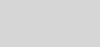# Format Multilevel Arrays in PowerShellDoctor Scripto

Summary: Microsoft Scripting Guy Ed Wilson talks about formatting multilevel arrays in Windows PowerShell.Hey, Scripting Guy! I am having a problem with arrays. I have two-level arrays and they work perfectly when I have the arrays defined on a single line. But when I try to format my script so that it is easier to read, they seem to get messed up. Can you help me?

—DFHello DF,

Microsoft Scripting Guy Ed Wilson here. Knowing how to work with arrays is fundamental to nearly all programing languages—at least the ones I know about. In Windows PowerShell, we have removed much of the mystery surrounding arrays and basically hidden the complexity. It is common to use @() to create an array, as shown here:

\$b = @(1,2,3)

But using @() is not required. For example, I can easily create an array by assigning more than one item to a variable. This technique is shown here:

\$a = 1,2,3

To display the contents of a variable that contains an array, call that variable. To access a specific element in an array, use square brackets to reference the element. These techniques are shown here:

PS C:\> \$a

1

2

3

PS C:\> \$a

1

PS C:\> \$a

3

If I want to add an extra element to an array, I use the += operator (think of it as adding an item, I want the variable containing the array, and then I make the variable containing the array equal to the new value). The following code illustrates this technique:

PS C:\> \$a += 6

PS C:\> \$a

1

2

3

6

All of these techniques are illustrated in the following figure.I can easily create an array that contains an additional array inside one of the elements. For instance, if I want to store the array that is contained in the variable \$a along with another array in a variable \$b, I can use the following technique:

\$a = 1,2,3

\$b = \$a,@(11,12,13)

If I look at what is stored in \$b, it is not at first obvious that it comprises two different arrays. This is shown here:

PS C:\> \$b

1

2

3

11

12

13

However, I can use square bracket notation and view the array stored in element 1 of the \$b variable:

PS C:\> \$b

11

12

13

I can also access each element of the array:

PS C:\> \$b

11

Storing arrays inside arrays in Windows PowerShell is both powerful and very easy to do. When writing a script, however, formatting this can become a problem. The following is one long command to store multiple arrays in the various elements of an array.

\$a = @(0,1,2,3),@(10,11,12,13),@(20,21,22,23),@(30,31,32,33),@(40,41,42,43),@(50,51,52,53)

As shown in the following figure, the multiple dimensions of the array are accessed via square brackets.DF, in the code you sent to me, you attempt to create another array around the various elements of arrays you have. But when the code runs, it does not work. Here is the code you sent:

\$a = @(@(0,1,2,3)

,@(10,11,12,13)

,@(20,21,22,23)

,@(30,31,32,33)

,@(40,41,42,43)

,@(50,51,52,53))

When the code runs and I attempt to index into the various elements of the array, the results are munged.

It is not necessary to surround the array with another @(). The change is rather simple. Just move the commas to the right, and it will work. This is shown in the revised code here:

\$a = @(0,1,2,3),

@(10,11,12,13),

@(20,21,22,23),

@(30,31,32,33),

@(40,41,42,43),

@(50,51,52,53)

When I run the code, I am able to index into the arrays as shown in the following figure.When dealing with the necessity of attempting to store multiple arrays into a single array, one pretty good approach is to store those arrays into variables, and then use the variables to build up the new array. This approach is shown here

\$b = 0,1,2,3

\$c = 10,11,12,13

\$d = 20,21,22,23

\$e = 30,31,32,33

\$f = 40,41,42,43

\$g = 50,51,52,53

\$a =  \$b,\$c,\$d,\$e,\$f,\$g

When the script runs, the output shown in the following figure is displayed.Well, DF, that is about all there is to working with and formatting multilevel arrays.

I invite you to follow me on Twitter and Facebook. If you have any questions, send email to me at scripter@microsoft.com, or post your questions on the Official Scripting Guys Forum. See you tomorrow. Until then, peace.

Ed Wilson, Microsoft Scripting Guy